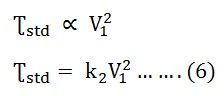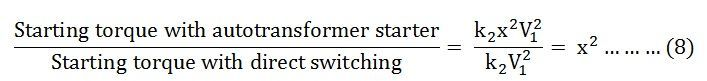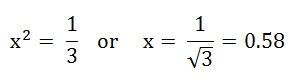# Autotransformer Starter

An Autotransformer Starter is suitable for both star and delta connected motors. In this method, the starting current is limited by using a three-phase autotransformer to reduce the initial stator applied voltage. The figure below shows the motor with the autotransformer starter:It is provided with a number of tappings. The starter is connected to one particular tapping to obtain the most suitable starting voltage. A double-throw switch S is used to connect the autotransformer in the circuit for starting. When the handle H of the switch S is in the START position, the primary of the autotransformer is connected to the supply line, and the motor is connected to the secondary of the autotransformer.

When the motor picks up the speed of about 80 percent of its rated value, the handle H is quickly moved to the RUN position. Thus, the autotransformer is disconnected from the circuit, and the motor is directly connected to the line and achieves its full rated voltage. The handle is held in the RUN position by the under voltage relay.

If the supply voltage fails or falls below a certain value, the handle is released and returns to the OFF position. Thermal overload relays provide overload protection whenever required.

## Theory of Autotransformer Starter

Figure (a) shown below represents the condition when the motor is directly switched on to lines and figure (b) shows when the motor is started with the help of an autotransformer.Let,

• Ze10 is the equivalent standstill impedance per phase of the motor referred to the stator side
• V1 is the supply voltage per phase.

When the full voltage V1 per phase is applied to the direct switching, the starting current drawn from the supply is given by the equation shown below:With auto transformer starting, if tapping of the transformer ratio x is used, then the voltage per phase across the motor is xV1. Therefore, at the starting, the motor current is given by the equation:In a transformer, the ratio of currents is inversely proportional to the voltage ratio provided that the no-load current is neglected. i.e.,If I’stl is the current taken from the supply by the autotransformer. Then,Substituting the value of Istm from the equation (2) in equation (3) we get.Since the torque developed is proportional to the square of the applied voltage, the starting torque with the direct switching is given as:Similarly, starting torque with autotransformer starterTherefore,With the autotransformer, at the starting, the motor current is given by the equation shown below:From the equation (3) and (9) we can conclude that,From the above equation (5) we get,Here equation (5) and equation (8) show that with an autotransformer, the starting current I’stl from the main supply and the starting torque is reduced to the x2 times to the corresponding values with the direct online starting.

Now, comparing equation (4) and equation (11) we get,Thus, the star delta starter is equivalent to an autotransformer starter of the ratio x = 0.58. A Star Delta starter is much cheaper than an autotransformer starter and is commonly used for both small and the medium size motors.

### 9 thoughts on “Autotransformer Starter”

1. i still Dont understand.Can someone elibrate for me please.Why we needed to install auto transformer

2. but if we are using auto transformer than input power is equal to output power amd if we reducing output voltage by xtimes than current should increase xtimes so that power remains constant ?
can u expalin this a little bit

3. Why we do use auto transformer starter when there is a cheap alternative, star-delta starter is also available.